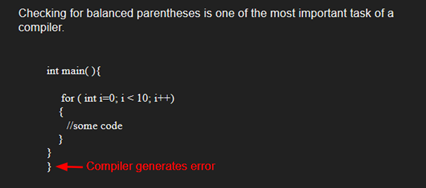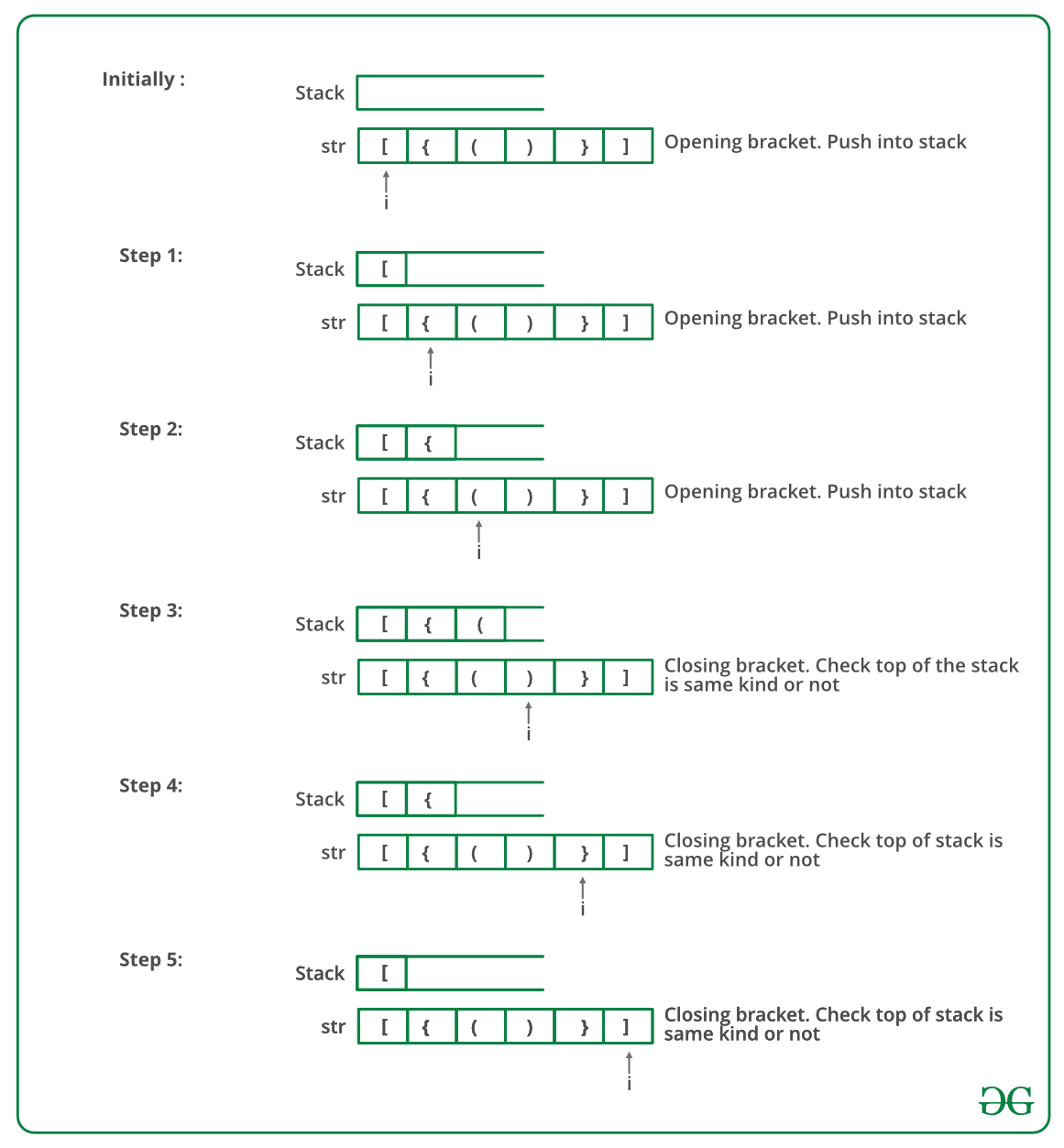# Java Program To Check For Balanced Brackets In An Expression (Well-Formedness) Using Stack

• Last Updated : 14 Dec, 2021

Given an expression string exp, write a program to examine whether the pairs and the orders of “{“, “}”, “(“, “)”, “[“, “]” are correct in exp.

Example

Input: exp = “[()]{}{[()()]()}”
Output: Balanced

Input: exp = “[(])”
Output: Not BalancedAlgorithm:

• Declare a character stack S.
• Now traverse the expression string exp.
1. If the current character is a starting bracket (‘(‘ or ‘{‘ or ‘[‘) then push it to stack.
2. If the current character is a closing bracket (‘)’ or ‘}’ or ‘]’) then pop from stack and if the popped character is the matching starting bracket then fine else brackets are not balanced.
• After complete traversal, if there is some starting bracket left in stack then “not balanced”

Below image is a dry run of the above approach:Below is the implementation of the above approach:

## Java

 `// Java program for checking``// balanced brackets``import` `java.util.*;`` ` `public` `class` `BalancedBrackets {`` ` `    ``// function to check if brackets are balanced``    ``static` `boolean` `areBracketsBalanced(String expr)``    ``{``        ``// Using ArrayDeque is faster than using Stack class``        ``Deque stack``            ``= ``new` `ArrayDeque();`` ` `        ``// Traversing the Expression``        ``for` `(``int` `i = ``0``; i < expr.length(); i++) ``        ``{``            ``char` `x = expr.charAt(i);`` ` `            ``if` `(x == ``'('` `|| x == ``'['` `|| x == ``'{'``) ``            ``{``                ``// Push the element in the stack``                ``stack.push(x);``                ``continue``;``            ``}`` ` `            ``// If current character is not opening``            ``// bracket, then it must be closing. So stack``            ``// cannot be empty at this point.``            ``if` `(stack.isEmpty())``                ``return` `false``;``            ``char` `check;``            ``switch` `(x) {``            ``case` `')'``:``                ``check = stack.pop();``                ``if` `(check == ``'{'` `|| check == ``'['``)``                    ``return` `false``;``                ``break``;`` ` `            ``case` `'}'``:``                ``check = stack.pop();``                ``if` `(check == ``'('` `|| check == ``'['``)``                    ``return` `false``;``                ``break``;`` ` `            ``case` `']'``:``                ``check = stack.pop();``                ``if` `(check == ``'('` `|| check == ``'{'``)``                    ``return` `false``;``                ``break``;``            ``}``        ``}`` ` `        ``// Check Empty Stack``        ``return` `(stack.isEmpty());``    ``}`` ` `    ``// Driver code``    ``public` `static` `void` `main(String[] args)``    ``{``        ``String expr = ``"([{}])"``;`` ` `        ``// Function call``        ``if` `(areBracketsBalanced(expr))``            ``System.out.println(``"Balanced "``);``        ``else``            ``System.out.println(``"Not Balanced "``);``    ``}``}`
Output
`Balanced`

Time Complexity: O(n)
Auxiliary Space: O(n) for stack.

Please refer complete article on Check for Balanced Brackets in an expression (well-formedness) using Stack for more details!

My Personal Notes arrow_drop_up